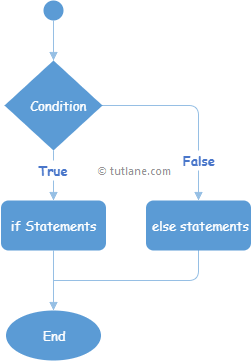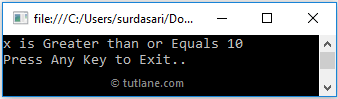# C# If Else Statement with Examples

In c#, if-else statement or condition has optional else statements, and these else statements will be executed whenever the if condition fails to execute.

Generally, in c# if-else statement, whenever the boolean expression returns true, the if statements will be executed otherwise, else block of statements will be executed.

## Syntax of C# if-else Statement

Following is the syntax of defining if-else statement in c# programming language.

if (boolean_expression)
{
// Statements to Execute if boolean expression is True
}
else {
// Statements to Execute if boolean expression is False
}

If you observe the above if-else statement syntax, the statements inside the if condition will be executed only when the “bool_expression” returns true otherwise, the statements inside of the else condition will be executed.

Following is the simple example of using the if-else statement in the c# programming language.

int x = 20;
if (x >= 10)
{
Console.WriteLine("x is Greater than or equals 10");
}
else
{
Console.WriteLine("x is less than or equals to 10");
}

If you observe the above example, whenever the defined condition (x >= 10) returns true, the if condition will be executed; otherwise, the else block of code will be executed.

## C# If-Else Statement Flow Chart Diagram

The following flow chart diagram will represent the process flow of the c# programming language's if-else statement.If you observe the above c# if-else statement flow chart, if the defined condition is true, then the statements within the if condition will be executed; otherwise, the statements inside the else block will be executed.

## C# If-Else Statement Example

Following is the example of defining an if-else statement in c# programming language to execute the block of code or statements based on a Boolean expression.

using System;

namespace Tutlane
{
class Program
{
static void Main(string[] args)
{
int x = 20;
if (x >= 10)
{
Console.WriteLine("x is Greater than or Equals to 10");
}
else
{
Console.WriteLine("x is Less than 10");
}
Console.WriteLine("Press Enter Key to Exit..");
}
}
}

If you observe the above code, we defined if-else conditions to execute the statements based on defined condition status, either true or false.

## Output of C# If Else Statement Example

When we execute the above c# program, we will get the result as shown below.If you observe the above result, the Boolean expression returns true that’s the reason only if block of statements has been executed and printed the statements in the console window.

This is how we can use the if-else statement in the c# programming language to execute the block of code or statements based on our requirements.